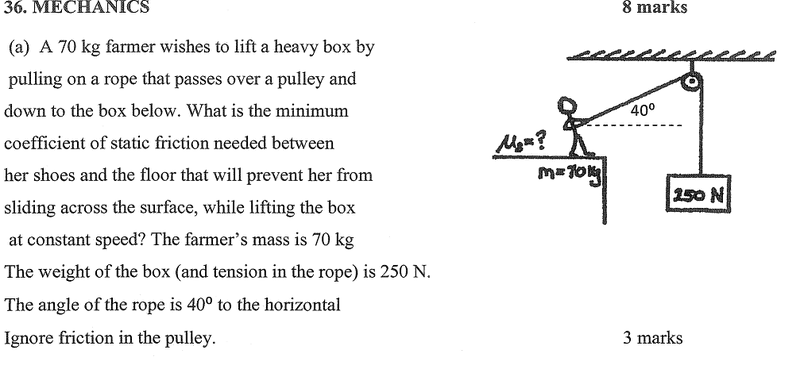# Mechanics - Pulley system finding coefficient of friction

## Homework Statement## Homework Equations

I believe:
F = m . a
Ffriction = μs . N
Ffriction = μs . mg . cosθ

## The Attempt at a Solution

I don't quite get how the equations above would give me an answer to the minimum friction required so that the farmer doesn't slip. I know Fl = (70x9.81)cos(40) = 526N But there is now a variable Ffriction that I don't know how to find...

I'm terrible at physics. If someone could explain or give me a head start, it would be appreciated.

## Answers and Replies

Hi Roaku!! Welcome to PFStart by making the free body diagram of the problem. You will have the forces balanced as, for the vertical direction, of the farmer...

$$Tsin\theta - mg = N$$

where N is the normal reaction, and T is tension in the rope.

Similarly, you will have an equation for the horizontal direction for the farmer, involving frictional force. Find the normal reaction from the above equation and use it in the one you made to get the minimum static friction.

## Homework Statement

View attachment 48197

## Homework Equations

I believe:
F = m . a
Ffriction = μs . N General Equatiom
Ffriction = μs . mg . cosθ NOT general equation

## The Attempt at a Solution

I don't quite get how the equations above would give me an answer to the minimum friction required so that the farmer doesn't slip. I know Fl = (70x9.81)cos(40) = 526N But there is now a variable Ffriction that I don't know how to find...

I'm terrible at physics. If someone could explain or give me a head start, it would be appreciated.

Use only general equation.
Then find value of N.
You also need to know not only the magnitude but also the direction of all the forces involve.

Last edited:
ehild
Homework Helper
Start by making the free body diagram of the problem. You will have the forces balanced as, for the vertical direction, of the farmer...

$$Tsin\theta - mg = N$$

where N is the normal reaction, and T is tension in the rope.

The free-body diagram refers to the man. N is the normal force exerted on his feet by the ground. It points upward. The ground can not exert downward force. The vertical component of the tension also points upward. The weight of the man points downward. So the equation for the vertical force components is Tsin(theta)+N-mg=0, that is, N= mg-Tsin(theta)

ehild

Whoops, I mis-posted the equation.

$$Tsin\theta - mg + N = 0$$

Thanks for observing that, ehild